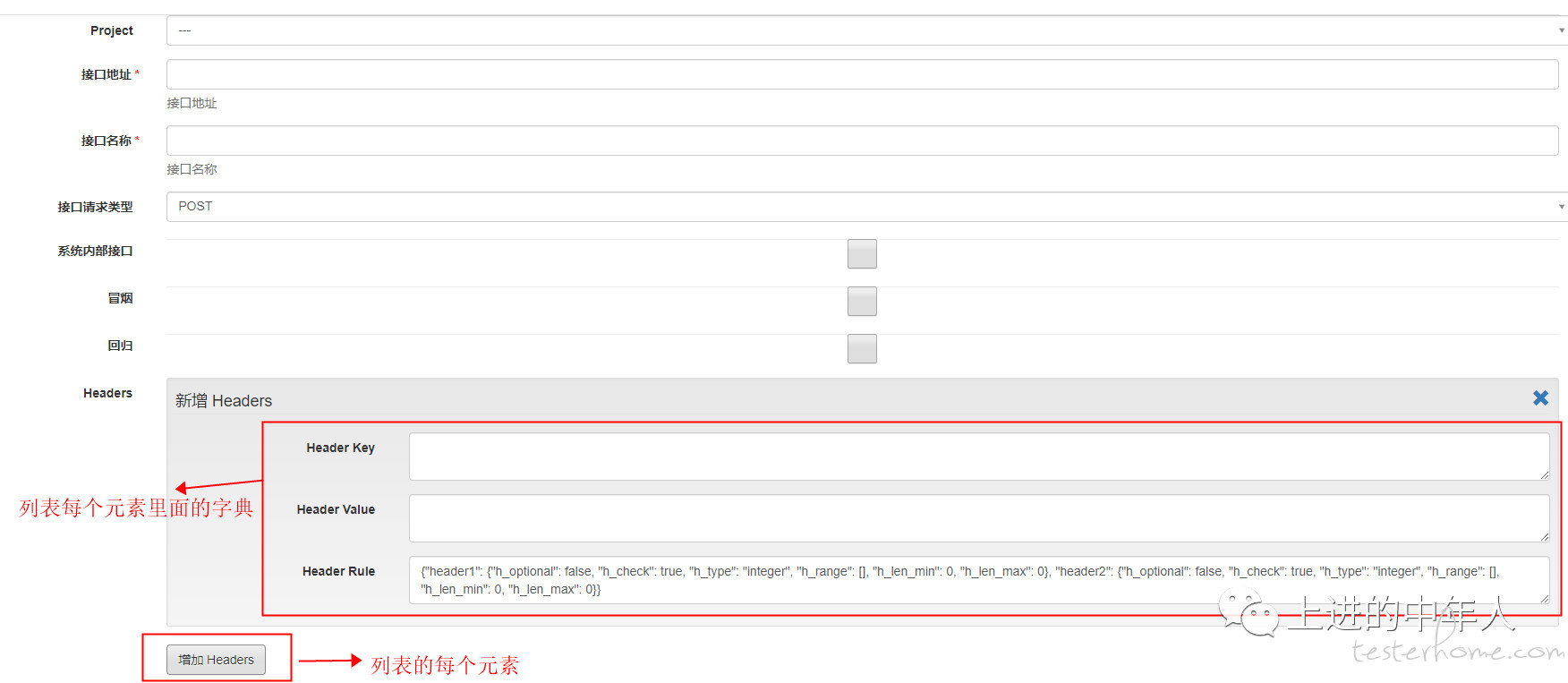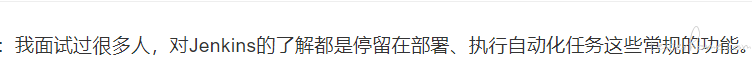### 框架的选型

``class Header(EmbeddedDocument):    header_key = StringField(db_field="header_key")    header_value = StringField(db_field="header_value")    header_rule = DictField(db_field="header_rule", description=u"规则",                            default={                                "header1": {"h_optional": False, "h_check": True, "h_type": "integer", "h_range": [],                                            "h_len_min": 0, "h_len_max": 0},                                "header2": {"h_optional": False, "h_check": True, "h_type": "integer", "h_range": [],                                            "h_len_min": 0, "h_len_max": 0}})class Data(EmbeddedDocument):    data_key = StringField(db_field="data_key")    data_value = StringField(db_field="data_value")    data_rule = DictField(db_field="data_rule", description=u"规则",                          default={"body1": {"b_default": "", "b_optional": False, "b_check": True, "b_type": "integer",                                             "b_range": [],                                             "b_len_min": 0, "b_len_max": 0},                                   "body2": {"b_default": "", "b_optional": False, "b_check": True, "b_type": "integer",                                             "b_range": [],                                             "b_len_min": 0, "b_len_max": 0}})class Asset(EmbeddedDocument):    asset_key = StringField(db_field="asset_key")    asset_value = StringField(db_field="asset_value")class InterfaceTestCaseForm(Document):    project = ReferenceField(ProjectForm)    creator = ReferenceField(TesterForm)    updater = ReferenceField(TesterForm)    interface = StringField(db_field="interface", description=u"接口地址",                            validators=[DataRequired(message=u"接口地址不能为空")], max_length=100)    name = StringField(db_field="name", description=u"接口名称",                       validators=[DataRequired(message=u"接口名称不能为空")], max_length=50)    interfaceType = StringField(db_field="interfaceType", description=u"接口请求类型",                                validators=[DataRequired(message=u"接口请求类型不能为空")], max_length=50)    testPlanType = ReferenceField(TestPlanTypeForm, db_field="testPlanType")    internal = BooleanField(db_field="internal", default=True)    smoke = BooleanField(db_field="smoke", default=True)    regression = BooleanField(db_field="regression", default=True)    testResult = StringField(db_field="testResult")    headers = ListField(        EmbeddedDocumentField(Header),        max_length=30)    data = ListField(        EmbeddedDocumentField(Data),        max_length=30)    asset = ListField(        EmbeddedDocumentField(Asset),        max_length=30)    createTime = StringField(db_field='createTime', max_length=20)  # TODO 修改改成DateField格式，参照TEST-57    updateTime = StringField(db_field='updateTime', max_length=20)  # TODO 修改改成DateField格式，参照TEST-57    deleted = BooleanField(db_field="deleted", default=False)    meta = {        "collection": "interfaceTestCase",        'ordering': ['-updateTime']    }``### 数据库选型

• mongo： 测试平台更多的与测试相关，处理各种json数据，编写各种接口请求等。所以主要用到的就是Mongo数据库，还有另外一个原因就是mongo的collection*不需要提前创建，不需要*定义任何字段类型，只需要代码里面定义即可，简直不要太方便（如下图）：
``class ProjectForm(Document):    projectName = StringField(db_field="projectName", validators=[DataRequired(message=u"项目名不能为空")], )    projectPrefix = StringField(db_field="projectPrefix", validators=[DataRequired(message=u"项目前缀,请与testlink保持一致")])    projectTestOwner = ReferenceField(TesterForm)    projectTesters = ListField(ReferenceField(TesterForm))  # 增加项目测试人员    projectDomain = StringField(db_field="projectDomain", validators=[DataRequired(message=u"项目域名不能为空")], )    projectAuthUrl = StringField(db_field="projectAuthUrl", validators=[DataRequired(message=u"系统登录地址不能为空")], )    authUser = StringField(db_field="authUser", validators=[DataRequired(message=u"系统默认登录用户名不能为空")], )    authPassword = StringField(db_field="authPassword", validators=[DataRequired(message=u"系统默认登录密码不能为空")], )    projectDescription = StringField(db_field="projectDescription", validators=[DataRequired(message=u"项目描述不能为空")])    meta = {        "collection": "projects"    }    def __unicode__(self):  # 解决对象引用过程中，前端看到的是可视化的名字，而不是对象名称。        return self.projectName``

• Redis：这里我没有选择Redis的原因只有一个，让平台简洁化。Redis能够实现的功能，如内存存储、锁，完全可以用Mongo和环境变量来替代。这里要讲一下Redis能够作为锁的主要原因是Redis是单进程的，可以创建一个对象，但是同一时间只有一个进程操作这个对象，也就实现了锁的功效。实战过程中会教大家如何用环境变量来实现锁的功能。

### 其他相关联的工具

• Jenkins：我面试过很多人，对Jenkins的了解都是停留在部署、执行自动化任务这些常规的功能。殊不知，Jenkins能实现的功能完全超乎你的想象，我曾经用Jenkins实现了一个外包人员的考核系统，用于考核外包人员对测试技能的掌握程度，这个功能的实现让我兴奋了一段时间。在这里，我用Jenkins主要是为了解决容器化部署带来的容器基础功能不完善以及测试平台一键部署问题。

• JIRA：JIRA作为常规的缺陷管理工具，自然成为测试平台需要集成的工具，包括各种数据报表的展示等。